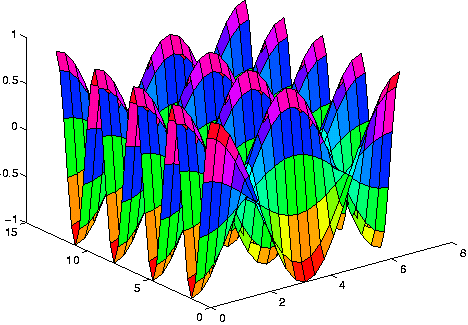MATLAB

(redirected from M-file)

MATLAB

(mathematics, language, application)
A high-level language and interactive program from The MathWorks for numeric computation and visualisation. MATLAB supports numerical analysis, matrix computation, signal processing, linear algebra, statistics, Fourier analysis, filtering, optimisation and numerical integration. It can output two and three dimensional graphics and can be integrated with C, C++, Fortran, Java, COM and Microsoft Excel.

http://mathworks.com/products/matlab/.

MATLAB

(MATrix LABoratory) A programming language for technical computing from The MathWorks, Natick, MA (www.mathworks.com). Used for a wide variety of scientific and engineering calculations, especially for automatic control and signal processing, MATLAB runs on Windows, Mac and a variety of Unix-based systems. Developed by Cleve Moler in the late 1970s and based on the original LINPACK and EISPACK FORTRAN libraries, it was initially used for factoring matrices and solving linear equations. Moler commercialized the product with two colleagues in 1984. MATLAB is also noted for its extensive graphics capabilities. The following MATLAB commands generate the 3D graph below:

```     x=(0:2*pi/20:2*pi)';
y=(0:4*pi/40:4*pi)';
[X,Y] = meshgrid(x,y);
z= cos(X).*cos(2*Y);
surf (x,y,z);
```

The Resulting GraphThis graph was computed from the lines of code above and is an example that might be used in multivariate calculus. (Image courtesy of Dr. Mark S. Gockenbach, Michigan Technological University, www.math.mtu.edu/~msgocken/intro/intro.html)
Mentioned in ?
References in periodicals archive ?
Parameters values of model's elements not specified in  are presented in the m-file listing (Fig.
M-file is used to enter the input values and automatically evaluate the total risk of the project.
Competitive Hunters Model (cancan m-file), (hunter m-file) (ode45 m-file for Octave) (cancan.
Haciendo uso de los valores del cuadro 1, en la figura 1, se presenta el script del M-file Quadratura.
20 allows engineers to use Math Script to integrate m-file scripts they created using the MATLAB development environment; create custom data acquisition devices with the new LabVIEW FPGA Wizard; and integrate third-party plant models from Dynasim and Plexim GmbH with the LabVIEW Simulation Module, a tool for designing and implementing control simulation models.
For the case of M-files, we approached the inter-procedural analysis problem by inlining all the M-files that are used in the program, including multiple levels of M-file calls (i.

Site: Follow: Share:
Open / Close